﻿ Azure Machine Learning – Episode 2 - Damir Dobric Posts - developers.de

# Azure Machine Learning – Episode 2

Damir Dobric Posts

#### Next talks:#### Syndication

In the first part (Episode 1) of this series I introduced Azure Machine Learning and problem domain which can be solved with this toolset. The I created the ne ML experiment and described how to deal with data sets.
In this part (episode 2) I will step-through an experiment, which should predict if the income will be greater or less than 50k, by using of real data published by US government.

### Selecting algorithm and training the model

As next we have to split dataset to data which will be used for training on to data which will be used to test/validate trained model. To do this we will use Split-shape.
In my example I will use 80% of data fro training and 20% for model validation. You can take a look in more options on the stencil if you want to peek some more specific data by using of regular expressions etc.
Usually, using of simple split is good enough.

After we split the data we need to chose the model used for learning. If you type “train” in the search box you will get all supported train models: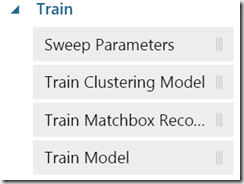Depending on problem which you are solving, different train approaches can be used. Currently supported approaches are not necessary provided as a theoretical constraint. Instead, they have more practical nature. For example “Train Matchbox Recommender” is a way to solve challenges in the interconnected world of the web, when people should be connected with other people, content, products etc..

In this example I will use more generalized “Train” approach. After dragging of the shape to the experiment we need to connect the Split-shape with the Train shape.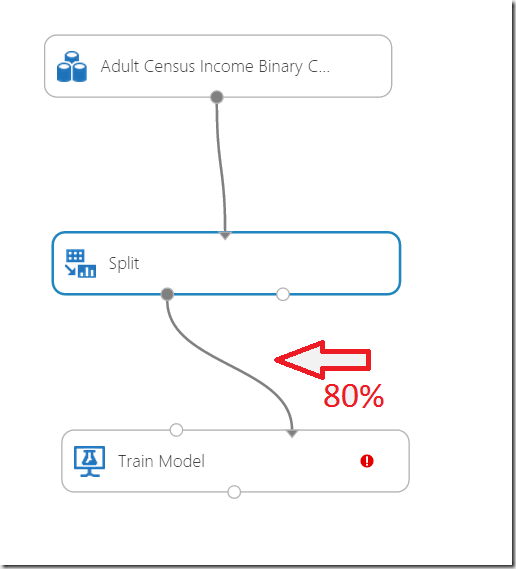The connect line basically means, that 80% of data (remember we split data to 0.8 + 0.2, where 0.8 will be used ass factor to randomly use 80% of data in dataset as training data. Connector must be connected to the right side of the shape.

The red indicator notifies that we didn’t select the column which has to be predicted. That is the column which we want to calculate. Remember our function:

income = f (age, workclass,..nativecountry)

So, we are going to calculate column “age”. By clicking on the right side to properties after we selected the Train Model shape, following dialog opens:

In the dialog we need to select the column “income”. After that all other columns will be used features (inputs in algorithm).

Sometimes, depending on dataset, we will not want to include all other columns in the calculation. In this case we would use Metadata Editor shape. This shape gives us a possibility to exactly chose columns which we need for calculation. In this example I will NOT use Metadata Editor, because my dataset exactly contains the data which I need.

#### Choosing Learning Algorithm

In the next step we need to chose the training algorithm. Navigate now to Machine Learning node under “Initialization” and notice following.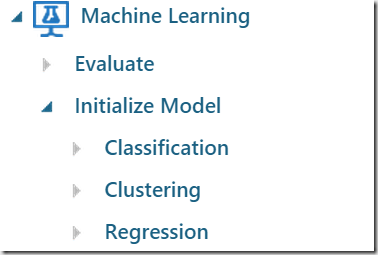There are 3 types of models: Classification, Clustering and Regression. Every of these types provides one or more different models. Because in our example we want to classify if somebody will earn more or less than 50k \$, we will use some classification algorithm. If we would have to calculate more exact income, then we would use Regression algorithm.

Commonly we will not know which algorithm is the best to solve some problem. But “experiment” is the IDE which enables us to try different algorithms and compare them, before we chose the final one. I selected “Two Class Logistic Regression”.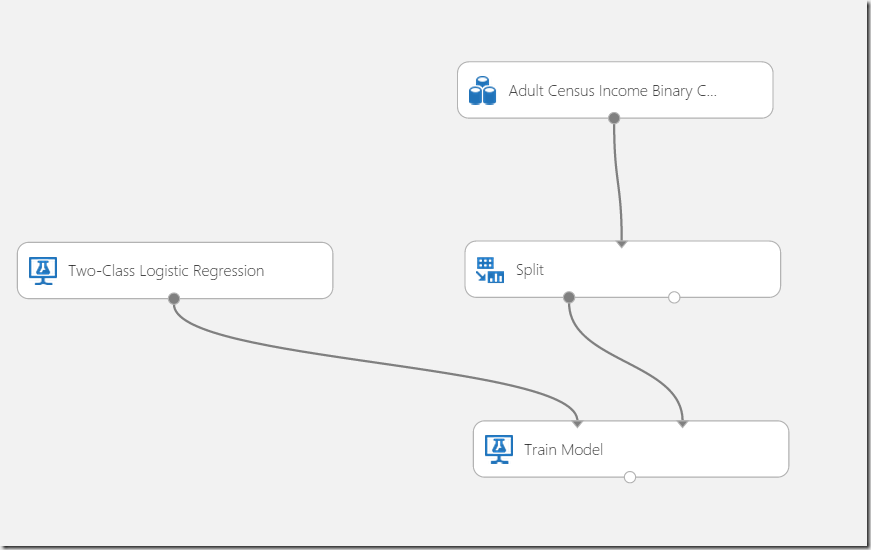This would be more or less all we need to train the system. Unfortunately the model shown on the picture above would work, but it wouldn’t gives us any result.
Typically every chosen algorithm will have a number of properties which can be used to tune the algorithm.
For example the logistic regression classifier used in this example has following properties, which can be shown when the shape is selected and properties clicked on the right side:

The winner question is “How do we know the meaning of this properties”? Here is a short overview of meaning of named variables related for algorithm used in this post (copy form online documentation).

• Optimization Tolerance

This parameter sets the threshold for the optimizer convergence. In other words, if the improvement between iterations is less than the threshold, the algorithm stops and returns the current model. The default value of this parameter is 1E-07.

• L1 Weight, L2 Weight

This learner can use a linear combination of L1 and L2 regularizations. The default value for both coefficients is 1.

• Memory Size

The technique used for optimization here is L-BFGS, which uses only a limited amount of memory to compute the next step direction. This parameter indicates the number of past positions and gradients to store for the computation of the next step. The default value of this parameter is 20.

In general ML documentation should help you to answer this question. Here are few URL-s which holds documentation related to algorithms:

Algorithms
Here you can find additional URLS for all algorithms.
http://help.azureml.net/Content/html/0C67013C-BFBC-428B-87F3-F552D8DD41F6.htm

Multiclass Logistic Regression
http://help.azureml.net/Content/html/1D195FE5-98CD-4E1A-A1C8-076AAA3E02C3.htm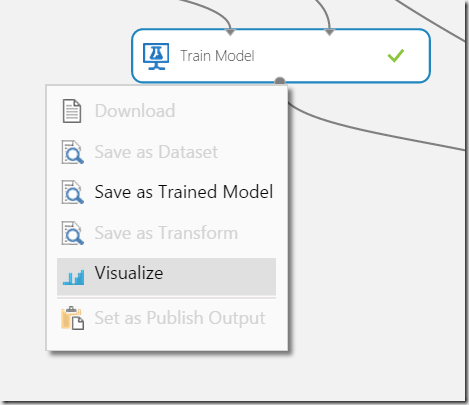In this case we will get few more information additionally to described algorithm properties. In this case we get additionally “User Threads” and “Allow Unknown Levels”. Without of digging deeper, all these values will be documented in the referenced documentation.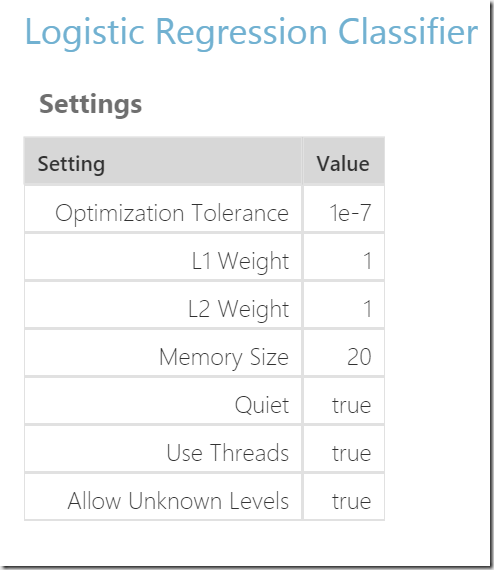But to make the model more useful, we need few more shapes. As next we will score the model.

#### Scoring

As next the trained model should be scored. To do that, lookup the Score Model shape and drag it to the experiment. Then connect the output of “Train Model” shape with the left input of the “Score Model” shape.
This connection represent result flow into the score model shape. The score model shape has also a second input. This is the input for data from data set which will be used for scoring. We will use (remember 20% in split shape on the beginning of this article series) 20% of data in dataset to test trained model. This is what second input does mean.
The picture below shows how the score model shape should be connected. Note that the score model has no any property which have to be set. All you have to do is to connect it to the output of the train model and test data provided by Split shape.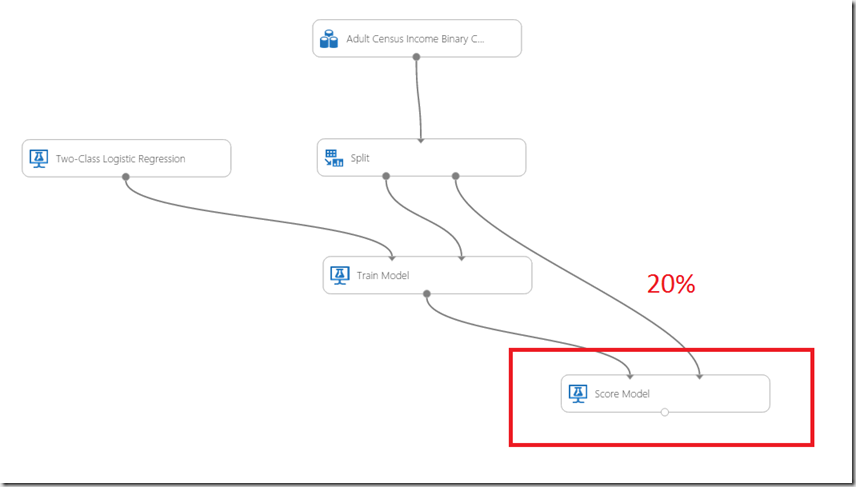No it is time to run the experiment. After click on run, notice the icon on the top-right, which indicates the request was queued. Depending on the dataset size you can follow, how shapes are executed step by step.
Please do not expect that everything here behaves sequentially as you expect. This model runs a way how data scientists expect it to run and not how developers would expect it. Anyhow after a while you will notice that status has changed from “Queued” to “Finished Running”After successful run you can visual score results (Click on output of the score model and then select visualize). First thing to notice is a number of rows 6512 which is exactly 20% of 32561, which is number of rows of dataset, which we used in this experiment. Note we used 20% of data for testing and 80% for training.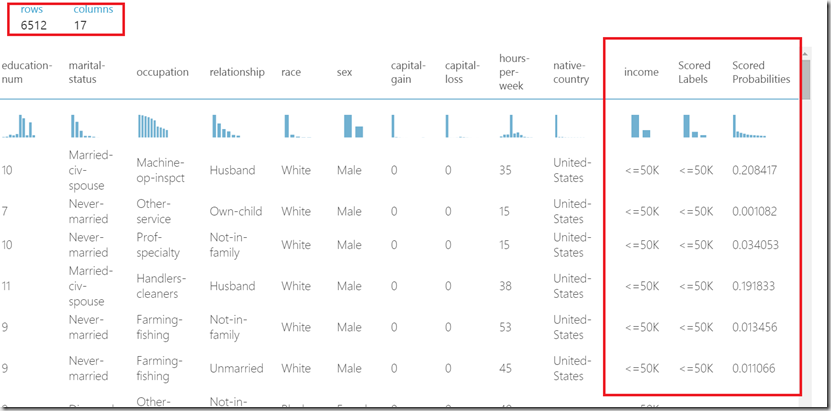It is even more interesting, that dataset shown at the picture above has two more columns than the original dataset. This are “Scored Labels” and “Scored Probabilities”.

“Scored Labels” is calculated result which indicates what the algorithm has calculated. In an ideal case this would always be same as value of column “income”. In reality the algorithm is mostly not perfect.
If you scroll down in the table shown above, you can find rows like following one: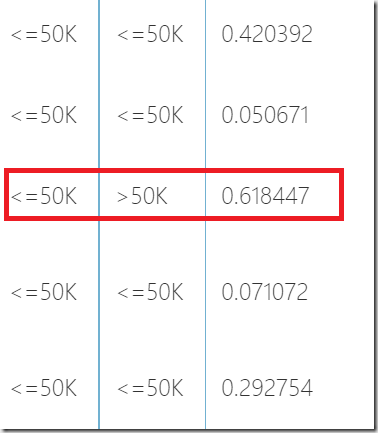The red marked row shows a mistake in learning process. The marked row was calculated as >50k, but expected value was <=50k. This case is called “true negative” and it is a cause of skepticism by those who do not feel that ML might be practically widely applicable.
How bad is the wrong guess in this case in another story. However this depends on statistic. If wrong guess happen in 10% cases, the algorithm cannot be accepted as very accurate.But imagine if this wrong guess would be the only on of 6512 examples. If that would be a machine with crashes one airplane of 6512 flights that would be very bad. But if this would be machine which guess movies or songs which you like, it would be more than acceptable.
This is also a question which I’m often asking myself. But what do you think in how many cases one doctor guess wrong antibiotic, or in how many cases one nation vote for wrong leader?
Interestingly it seems that voting a wrong leader does not feel that bas as crashing of a single airplane.
But, if you are still skeptic I would recommend to take a look on great session by Professor on Caltech Yaser Abu-Mostafa. He explained mathematically that learning is feasible. This is what he declared as a “final verdict”“Score Probabilities” is a real value, which represents the calculated probability that income is below or over the 50k. Values less than 0.5 will set the scored label income to <-50k. All values grater than 0.5 will set scored label income to >50k.

Getting more from data

When looking on scored label, we can always learn even more from data. For example, take a look on histogram, we figure out that there are nearby 500 scores, which have none valid value “null”.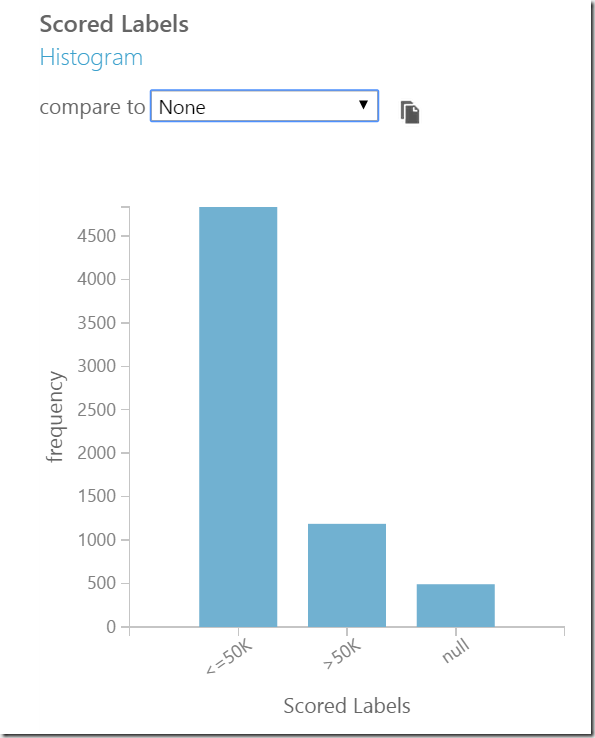Such cases ware not calculated, because typically at leas one of features was missing. Following picture show such example. Because the country was missing the algorithm was not able to calculate income.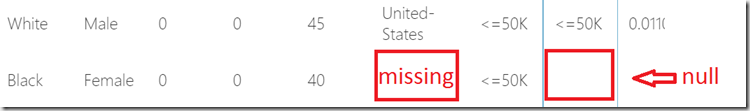If we now compare scored income (scored labels) with real income we get following cross-tab: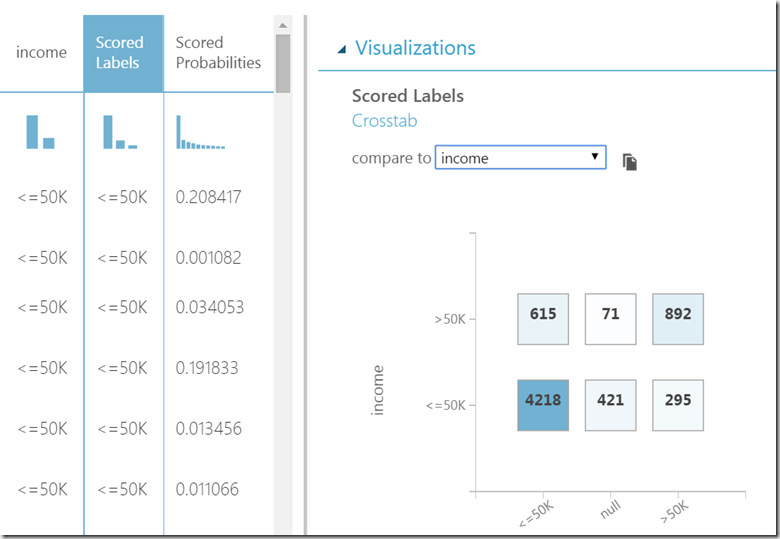In 4218 case guessed income of <=-50k matches the real income. This is called TRUE NEGATIVES. In 892 cases we guess correctly income >-50k. This is called TRUE POSITIVES.
We also have 421+71 = 492 null results without of meaning, because the data was fully provided.
Unfortunately we also have 615 incorrect guesses. For income >50k algorithm calculated income <=50k. This is called FALSE NEGATIVES. Similarly we have 295 FALSE POSITIVES. Perfect algorithm would have zero NAGATIVES.

TRUE – guess is correct
FALSE-guess is incorrect
POSITIVES – Related to values greater than threshold (>50k)
NEGATIVES – Related to values less than threshold (<50k)

True Positive

True Positive (TP):  Healthy people correctly identified as healthy

True Negative

True Negative (TN):  Sick people correctly diagnosed as sick

False Positive

False Positive (FP): Sick people incorrectly identified as healthy

False Negative

False Negative (FN): Healthy people incorrectly identified as sick

To understand this: sick is negative and health is positive. true is correct guess and false is incorrect guess.

#### Model Evaluation

If we want to get more from data it is helpful to use Evaluate Model shape.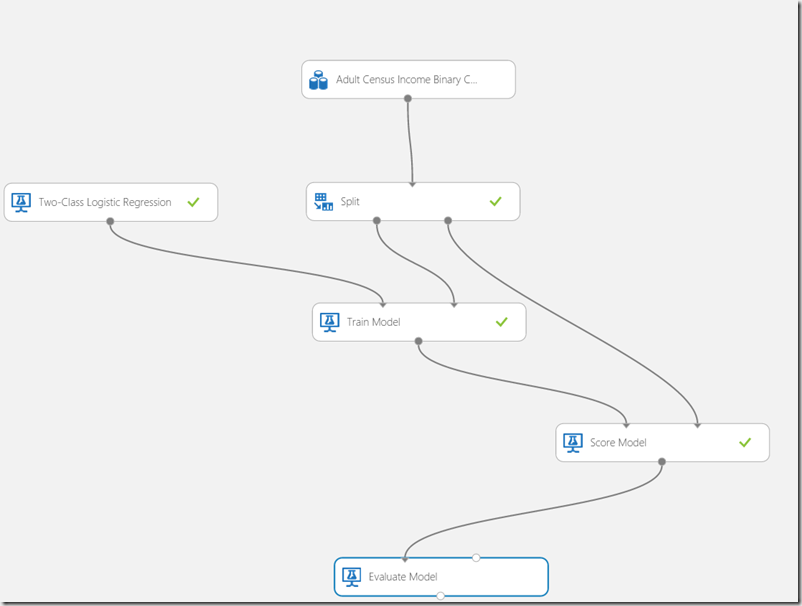Simply drag the shape to experiment and connect it with the Score Model and run experiment again. Right now evaluation process distinguish between Regression Model and Classification Model. depending on model evaluation will generate different metrics.

Regression

Following metrics are used in regression evaluator.
Mean Absolute Error(MAE) measures how close are predictive and true outcomes to each other.Whereis the prediction andthe true value

Root Mean Squared Error (RMSE) is similar to MAE, but with root square error calculation.Whereis the prediction andthe true value

Relative Absolute Error (RAE)Whereis the prediction andthe true value andis the mean ofRelative Squared Error (RSE)Whereis the prediction andthe true value andis the mean ofCoefficient Determination##### Classification

Accuracy

The proportion of the total number of correct predictions.

Accuracy = (TP + TN) / (TP + TN + FP + FN)

Precision

Precision = TP / (TP + FP)

Recall

Recall = TP / (TP + TN)

F1 Score

F1 Score is the harmonic mean of precision and Recall.

F1 = 2TP / (2TP + FP + FN)

Threshold

Threshold is the value above which it belongs to first class and all other values to the second class. E.g. if the threshold is 0.5 then any patient scored more than or equal to 0.5 is identified as sick else healthy.

#### References:

Short Video Series about Azure ML: